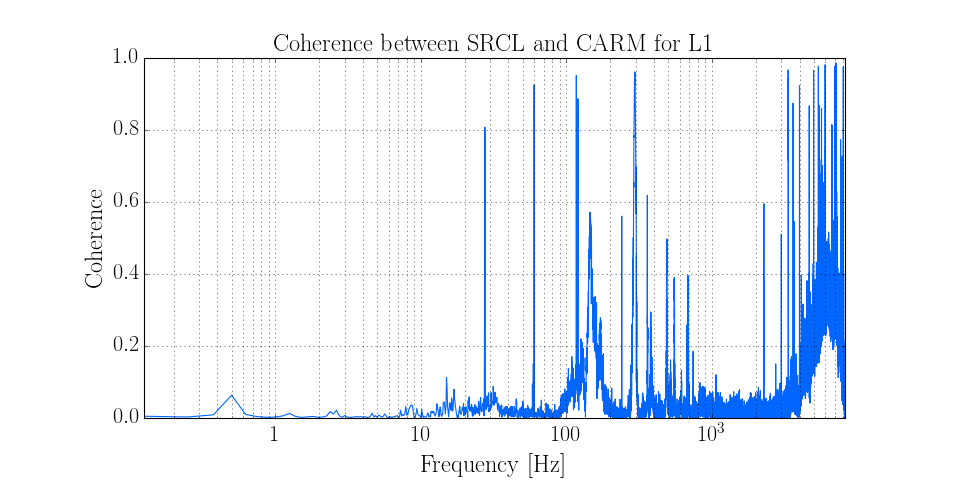# Calculating the coherence between two channels¶

The coherence between two channels is a measure of the frequency-domain correlation between their time-series data.

In LIGO, the coherence is a crucial indicator of how noise sources couple into the main differential arm-length readout.

In this example we calculate the coherence between two length-sensing degrees of freedom, the Signal-Recycling Cavity length (SRCL), and the Common-Arm motion (CARM).

First, we import the `TimeSeriesDict`

```from gwpy.timeseries import TimeSeriesDict
```

and then `get()` both data sets:

```data = TimeSeriesDict.get(['L1:LSC-SRCL_IN1_DQ', 'L1:LSC-CARM_IN1_DQ'],
'Feb 13 2015', 'Feb 13 2015 00:15')
```

We can then calculate the `coherence()` of one `TimeSeries` with respect to the other, using an 8-second Fourier transform length, with a 4-second (50%) overlap:

```coh = data['L1:LSC-SRCL_IN1_DQ'].coherence(data['L1:LSC-CARM_IN1_DQ'], 8, 4)
```

Finally, we can `plot()` the resulting data:

```plot = coh.plot(figsize=[12, 6], label=None)
ax = plot.gca()
ax.set_yscale('linear')
ax.set_xlabel('Frequency [Hz]')
ax.set_ylabel('Coherence')
ax.set_title('Coherence between SRCL and CARM for L1')
ax.grid(True, 'both', 'both')
plot.show()
```